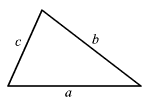# C Program to determine the type and Area of a Triangle

Last updated on July 27, 2020

The following is a C Program to determine the type and Area of a Triangle:

  1 2 3 4 5 6 7 8 9 10 11 12 13 14 15 16 17 18 19 20 21 22 23 24 25 26 27 28 29 30 31 32 33 34 35 36 37 38 39 40 41 42 43 44 45 46 47 48 49 50 51 52 53 54 55 56 57 58 59 60 /***************************************************** Program to determine the type and area of a triangle *****************************************************/ #include // include stdio.h library #include // include math.h library int main(void) { float a, b, c, s, area; printf("Enter sides of a triangle: \n"); printf("a: "); scanf("%f", &a); printf("b: "); scanf("%f", &b); printf("c: "); scanf("%f", &c); // sum of any two sides must be greater than the third side if( (a + b > c) && (b + c > a) && (c + a > b) ) { // three sides are equal if( (a == b) && (b == c) ) { printf("Triangle is equilateral.\n"); } // two sides are equal else if( (a == b) || (b == c) || (a == c) ) { printf("Triangle is isosceles.\n"); } // no sides are equal else { printf("Triangle is scalene.\n"); } // area of triangle using heron's formula https://en.wikipedia.org/wiki/Heron's_formula s = (a + b + c) / 2; //semi perimeter area = sqrt( s * (s - a) * (s - b) * (s - c) ); // area printf("Area of triangle %.2f.", area); } else { printf("Sides don't make a triangle."); } return 0; // return 0 to operating system } 

Expected Output:

1st run:

 1 2 3 4 5 6 Enter sides of a triangle: a: 3 b: 4 c: 5 Triangle is scalene. Area of triangle 6.00. 

2nd run:

 1 2 3 4 5 Enter sides of a triangle: a: 4 b: 5 c: 1 Sides don't make a triangle. 

## How it works #

The above program uses two theorems:

• Triangle Inequality Theorem
• Heron's Formula

### Triangle Inequality Theorem #

The Triangle Inequality Theorem states that the sum of two sides of a triangle must be greater than the third side.Let a, b c be the three sides of the triangle then according to Triangle Inequality theorem:

 1 2 3 a + b > c b + c > a c + a > b 

We can also use Triangle Inequality theorem to determine whether the given three line segments can be used to construct a triangle or not.

In order for three line segments to form the sides of a triangle, all the three conditions must be satisfied.

If any one of the condition fails then the given line segments can't be used to construct a triangle. For example:

Example 1: Can we construct a triangle using the following lengths: 7, 3, 2?

 1 2 3 7 + 3 > 2 => true 3 + 2 > 7 => false 7 + 2 > 3 => true 

The second condition is false. Hence, lengths 7,3 and 2 can't construct a triangle.

Example 2: Can we construct a triangle using the following lengths: 3, 4, 5?

 1 2 3 3 + 4 > 5 => true 4 + 5 > 3 => true 5 + 3 > 4 => true 

All the three conditions are true. Hence, lengths 3, 4, 5 can be used to construct a triangle.

## Heron's Formula #

Heron's Formula allows us to find the area of the triangle using the length of the three sides.

\begin{gather*}
Area=\sqrt{s(s-a)(s-b)(s-c)}
\end{gather*}

where s is called the semi perimeter of the triangle and is calculated as follows:

\begin{gather*}
s=\frac{a+b+c}{2}
\end{gather*}